Function Repository Resource:

# SpectralRandomnessTest

Use a discrete cosine transform–based method to test the randomness of a sequence of random reals

Contributed by: Emmy/Noah Blumenthal
noahb320@gmail.com
emmyb320@bu.edu
 ResourceFunction["SpectralRandomnessTest"][sequence] conducts a discrete cosine transform-based test to asses the randomness of either a sequence of 0s and 1s or reals between 0 and 1, returning the associated p-value. ResourceFunction["SpectralRandomnessTest"][sequence,"property"] conducts a discrete cosine transform-based test and returns the associated property.

## Details and Options

Properties include:
 "TestStatistic" returns the test statistic "PValue" returns the p-value associated with the test
The test statistic is generated by comparing the discrete cosine transformed data with the normal distribution using the Kolmogorov-Smirnov test.
The test works for sequences of of random reals between 0 and 1 and sequences of only 0s and 1s

## Examples

### Basic Examples (2)

Generate a sequence of random integers:

 In:=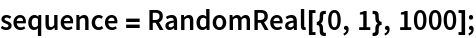Visualize the sequence:

 In:=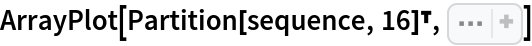Out=Apply a discrete cosine transform-based test:

 In:=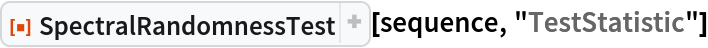Out=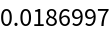In:=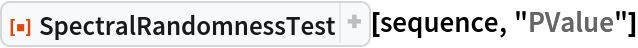Out=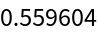Generate a sequence of random integers:

 In:=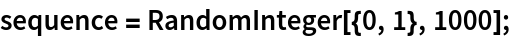Visualize the sequence:

 In:=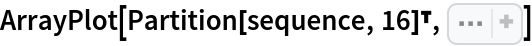Out=Apply a discrete cosine transform-based test:

 In:=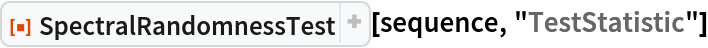Out=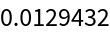In:=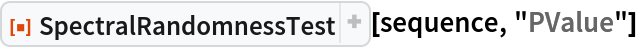Out=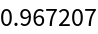### Scope (3)

Generate a sequence of random integers:

 In:=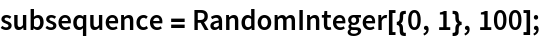In:=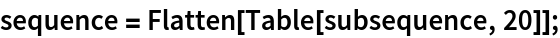Visualize the sequence:

 In:=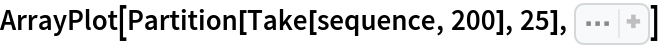Out=Attempt to reject a non-random sequence:

 In:=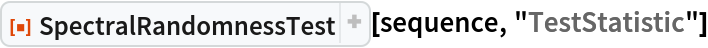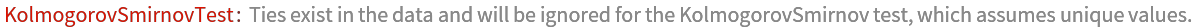Out=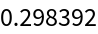In:=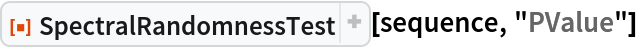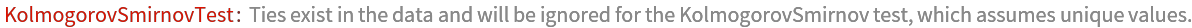Out=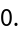### Applications (1)

Test to see if rule 30 is random according to the spectral test:

 In:=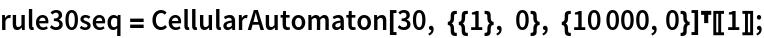In:=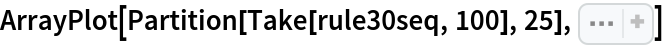Out=In:=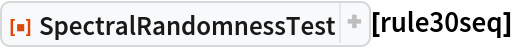Out=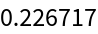### Possible Issues (1)

For non-random data, the Kolmogorov–Smirnov test, a part of the entire test, may return ties. Observing such ties is a strong indicator that the data is non-random:

 In:=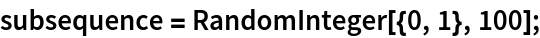In:=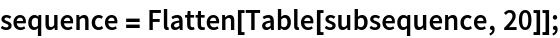In:=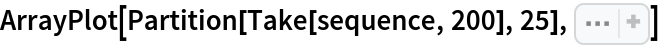Out=In:=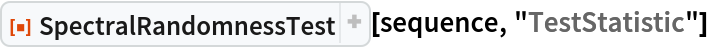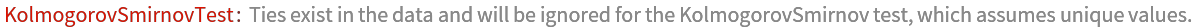Out=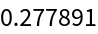In:=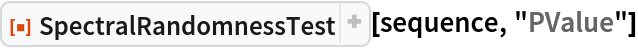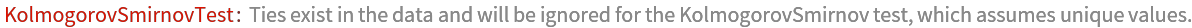Out=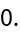### Neat Examples (1)

Visualize the sampling distribution of the test statistic:

 In:=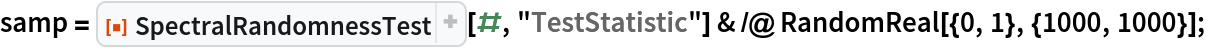In:=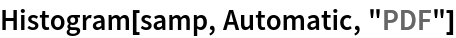Out=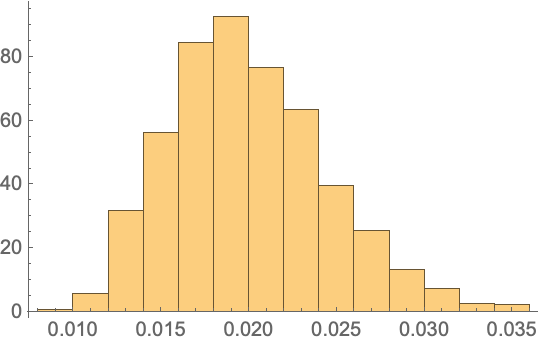Emmy Blumenthal

## Version History

• 1.0.0 – 08 July 2019